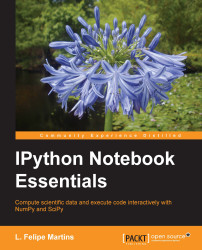•#### IPython Notebook Essentials#### Overview of this book

IPython Notebook EssentialsCreditswww.PacktPub.comPrefaceFree Chapter
A Tour of the IPython NotebookThe Notebook InterfaceGraphics with matplotlibHandling Data with pandasAdvanced Computing with SciPy, Numba, and NumbaProIPython Notebook Reference CardA Brief Review of PythonNumPy ArraysIndex## Accelerating computations with Numba and NumbaPro

In this section, we will discuss `Numba` and `NumbaPro`, two very exciting libraries to accelerate the `NumPy` code. `Numba` and `NumbaPro` were created by Continuum Analytics, the same company that produces the Anaconda distribution. `Numba` is part of the standard Anaconda distribution, but `NumbaPro` is a commercial product that must be purchased separately as part of the `Accelerate` package. However, `NumbaPro` can be downloaded for a free trial period.

These libraries are unique in that they allow the acceleration of code with the addition of a few lines of code. As the first example, let's consider the following lines of code to multiply two matrices:

```def matrix_multiply(A, B):
m, n = A.shape
n, r = B.shape
C = zeros((m, r), float64)
for i in range(m):
for j in range(r):
acc = 0
for k in range(n):
acc += A[i, k] * B[k, j]
C[i, j] = acc
return C
```

The preceding code uses the...